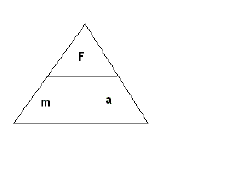#### Newton's Laws of Motion

Isaac Newton defined and described the motion of objects using three very simple but powerful Laws.

##### The First Law

This law is also known as the Law of Inertia.  Inertia is a measure of the "laziness" of an object; its resistance to movement or to the change of motion.

Newton's Law of Inertia states that an "Objects at rest will stay at rest or will continue to move in the same direction and with the same speed, unless an external (unbalanced) force acts upon them".

Example:
If you are a passenger in a car cruising at a steady, comfortable speed of say 60 km/h and suddenly the driver hits the brakes -- what will happen?

You will continue to move forward at 60 km/h because you have the tendency to keep doing what you were doing before the brakes were applied, i.e. move at a constant speed of 60 Km/h.  [Wear seatbelts next time you are in a car].

##### The Second Law

This law of motion looks at the force that will force objects to change their state of rest or motion.  In soccer, the harder you kick the ball, the faster in moves (accelerates).  The ball moves in the direction of the kick [provided you kick it dead on -- no funny moves].

If you kick a golf ball with the same force as the soccer ball -- what happens?

The golf ball will accelerate much faster -- why?

Because the golf ball has less mass than the soccer ball.

Newton's Second Law states that the stronger the force acting on a mass, the greater its acceleration.  If the mass increases, the acceleration will decrease if the force stays constant.

The acceleration of an object is proportional to the unbalanced force and is inversely proportional to the mass of the object.

In mathematical terms:

Force = mass X acceleration

F = m x a

Using Symbols......Use the above triangle as a memory aid to help you solve problems and to rearrange the basic equation F = ma to solve for the other two variables; a and m

##### The table below summarizes the units and quantities involved in the mathematical form of Newton's Second Law of Motion.
 Quantity Symbol Unit Force F Newton [N] Mass m Kilogram [Kg] Acceleration a m/s2

##### The Newton -- a unit of force
• 1 Newton [N] is approximately the force of gravity acting on a medium sized apple.
• 1 Newton is also defined as the force that accelerates a 1 Kg mass at 1 m/s.

Make sure that the units are always in Newtons, Kilograms, and meters-per-second-squared when this formula is used.

Note:

One Newton of force can also be defined as One  [Kilogram-meter-per-seconds-squared] ==> (Kg)(m)/s2

Example:  What is the force required to make a 300 g soccer ball accelerate at 2.0 m/s2  ?

Solution:

Given:  m = 300 g = 0.30 Kg
a   = 2.0 m/s

Find: F

Solution:  F = m X a
F = 0.30 Kg X 2.0 m/s2
= 0.60 N

##### The Third Law

This law of motion looks is also known as the action-reaction Law.

"For every action, there exists an equal but opposite reaction".

Examples:

• When rowing a boat one notices that the boat moves in the opposite direction of the motion of the oars.
• When you skate, you push back on the skates to move forward.
• The recoil action of a rifle: as the bullet leaves the muzzle of the gun, the gun kicks back in the opposite direction.